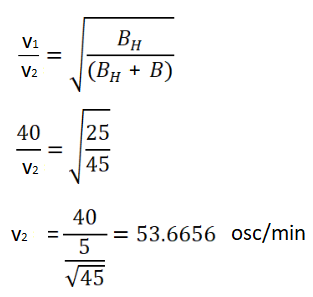# HC Verma Solutions Class 12 Chapter 14 Permanent Magnets

HC Verma Solutions Class 12 Chapter 14 Permanent Magnets will help students become better problem solvers and develop efficient analytical skills that will be helpful in tackling complex questions given in this chapter. Apart from that students will further gain a clearer understanding of concepts. The solutions provided here contain detailed answers to all the exercises which students can refer and study productively for the exams especially competitive ones like IIT JEE.

With all the topics, the HC Verma solutions will give students a good idea of tackling difficult numerical problems in the right manner and in less time.

Students can access the solution below.

## Key Concepts Related To Permanent Magnets

As for the chapter, it deals with the topic of magnets and related concepts like;

• Magnetic Poles and Bar Magnets
• Torque and Magnetic Field on a Bar Magnet
• Magnetic Scalar Potential
• Terrestrial Magnetism
• Determination of Dip
• Neutral Point
• Tangent and Moving Coil Galvanometer
• Shunt
• Tangent Law of Perpendicular Fields
• Deflection and Oscillation Magnometer
• Gauss’s Law for Magnetism

## Class 12 Important Questions In Chapter 14

1. Do you think that in a magnetic dipole two distinct poles can actually exist at two nearby points?

2. Is it possible for the earth’s magnetic field to be vertical at a place? How will a freely suspended magnet react at such places? Give the value of dip in such cases?

3. We take a tangent galvanometer and connect it to a battery. Now, if we double the number of turns in the coil what will happen to the deflection?

(a) no changes (b) it increases (c) decreases (d) it can either increase or decrease

4. State your observation when a compass needle is allowed to move in a horizontal plane in a geomagnetic pole.

(a) will stay in any position (b) there is no movement (c) will stay in an east-west direction (d) it will stay in the north-south direction

5. What is Magnetic meridian?

(a) It is a vertical plane (c) It is a horizontal plane (b) A line along north-south (d) Just a point

## HC Verma Solutions Vol 2 Permanent Magnets Chapter 14

Question 1: A long bar magnet has a pole strength of 10 Am. Find the magnetic field at a point on the axis of the magnet at a distance of 5 cm from the north pole of the magnet.

Solution:

M = 10Am

r = 5cm = 0.05m = 5 x 10-2 m

and μ0 = 1.257 x 10-6 henry per meter

The magnetic field due to magnetic charge B:

B = μ0/4π x M/r

B = [1.257×10-6]/[4×3.142] x 10/[(5×10-2)2]

B = 4 x 10-4 T

Question 2: Two long bar magnets are placed with their axes coinciding in such a way that the north pole of the first magnet is 2.0 cm from the south pole of the second. If both the magnets have a pole strength of 10 Am, find the force exerted by one magnet on the other.

Solution:

Let M1 and M2 are two poles.

M1 = M2 = 10 Am

and r2 = 2 cm = 2 x 10-2 m

Now,

Force exerted by one magnet of the other F:

F = μ0/4π x M1M2/r2

F = [1.257×10-6]/[4×3.142] x 102/(2×10-2)2

F = 2.5 x 10-2 N

Question 3: A uniform magnetic field of 0.20 × 10–3 T exists in the space. Find the change in the magnetic scalar potential as one moves through 50 cm along the field.

Solution:

Given: B = 0.20 × 10–3

and r = 50 cm = 50 x 10–2 m

Change in the magnetic scalar, ΔV

Here B = -dv/dl

dv = -B dl

Integrate above equation, we get

∫dv = -B ∫dl

From range r1 to r2

So, ΔV = B x Δr = -0.20 x 10–3 x 50 x 10–2

ΔV = -0.1 x 10–3

Question 4: Figure shows some of the equipotential surfaces of the magnetic scalar potential. Find the magnetic field B at a point in the region.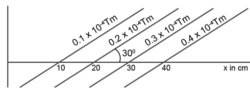Solution:

Change in potential = dv = 0.1 x 10–4 Tm

Perpendicular distance = dx = 10 sin 300 = 5 x 10-2 m

Relation between the potential and the field:

B = -dv/dx = -[0.1 x 10–4]/[5 x 10–2] = -2 x 10–4 T

B is perpendicular to equipotential surface. It is at angle of 120 degree with the +ve x-axis.

Question 5: The magnetic field at a point, 10 cm away from a magnetic dipole, is found to be 2.0 × 10–4 T. Find the magnetic moment of the dipole if the point is

(a) in end-on position of the dipole and

(b) in broadside-on position of the dipole.

Solution:

Here d = 10 cm = 10 x 10–2 m

and B = 2 x 10–4 T

(a) End-on-position:

B = μ0/4π x 2M/d3

2 x 10–4 = [10–7 x 2M]/[(10×10–2)3]

M = 1 A -m2

B = μ0/4π x M/d3

2 x 10–4 = [10–7 x M]/[(10×10–2)3]

M = 2 A-m2

Question 6: Show that the magnetic field at a point due to a magnetic dipole is perpendicular to the magnetic axis if the line joining the point with the centre of the dipole makes an angle of tan–1 (√2) with the magnetic axis.

Solution:

Let θ = tan–1 (√2)

tan θ = (√2) or tan2 θ = 2, can also written as cot θ = tanθ/2

Also, we know tanθ/2 = tan α

Equating above equations, we have

tan α = cot θ = tan(90o – θ)

or α = 90-θ

or θ + α =90°

The magnetic field at a point due to a magnetic dipole is perpendicular to the magnetic axis. Hence Proved.

Question 7: A bar magnet has a length of 8 cm. The magnetic field at a point at a distance 3 cm from the centre in the broadside-on position is found to be 4 × 10–6 T. Find the pole strength of the magnet.

Solution:

Length of the magnet = 2l = 8 cm = 8 x 10-2 m

Distance of observation point from the centre of dipole = d = 3 cm

Magnetic field due to the dipole on the equatorial point: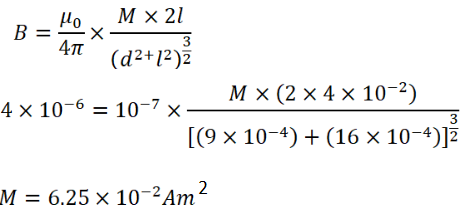Question 8: A magnetic dipole of magnetic moment 1.44 A m2 is placed horizontally with the north pole pointing towards north. Find the position of the neutral point if the horizontal component of the earth’s magnetic field is 18 μT.

Solution:

Here M = 1.44 Am2

We know that for a magnetic dipole with its pole facing north, the neutral point is always in the broadside position.

Magnetic field due to the dipole at the broadside-on position:

B = μ0M/4πd3

18 x 10-6 = [10-7 x 1.44]/d3

d = 0.2 m = 20 cm

Question 9: A magnetic dipole of magnetic moment 0.72 A m2 is placed horizontally with the north pole pointing towards south. Find the position of the neutral point if the horizontal component of the earth’s magnetic field is 18 μT.

Solution:

We know that for a magnetic dipole with its pole facing north, the neutral point is always in the broadside position.

B = μ0(2M)/4πd3

18 x 10-6 = [10-7x0.72]/d3

d = 0.2 m = 20 cm

Question 10: A magnetic dipole of magnetic moment 0.72√2 A m2 is placed horizontally with the north pole pointing towards east. Find the position of the neutral point if the horizontal component of the earth’s magnetic field is 18 μT.

Solution:

Horizontal component of the earth’s magnetic field = BH = 18 μT = 18×10-6 T

We know, B = μ0M/2πd3

At any point, B = BH

=> 18 x 10-6 = [10-7 x 0.72√2]/d3

d = 0.2 m = 20 cm

Question 11: The magnetic moment of the assumed dipole at the earth’s centre is 8.0 × 1022 Am2. Calculate the magnetic field B at the geomagnetic poles of the earth. Radius of the earth is 6400 km.

Solution:

We know, B = μ0 (2M)/4πd3

B = [10-7 x 2 x8x1022]/[(64)3x1015]

B = 6 x 10-5 T

Question 12: If the earth’s magnetic field has a magnitude 3.4 × 10–5 T at the magnetic equator of the earth, what would be its value at the earth’s geomagnetic poles?

Solution:

Magnetic field at the magnetic equator = B1 = 3.4 × 10–5 T

Since the point on the magnetic equator is at the equatorial position of the earth’s magnet, the magnetic field is at the equatorial point:

We know, B1 = μ0 (2M)/4πR3

3.4 x 10-5 = [10-7 x M]/R3

M = 3.4 x 102 R3

Now, magnetic field at the pole,

B2 = μ0 (2M)/4πd3

B2 = 10-7 x [2×3.4 x 102 R3]/R3

B2 = 6.8 x 10-5 T

Question 13: The magnetic field due to the earth has a horizontal component of 26 μT at a place where the dip is 60°. Find the vertical component and the magnitude of the field.

Solution:

The magnetic field due to the earth has a horizontal component = BH = 26 μT = 26×10-6 T

and θ = 60 degrees

For horizontal component:

BH = B cos 600

26×10-6 = B/2

=>B = 52 x 10-6 T

Or B = 52 μT

Question 14: A magnetic needle is free to rotate in a vertical plane which makes an angle of 60° with the magnetic meridian. If the needle stays in a direction making an angle of tan–1(2/√3) with the horizontal, what would be the dip at that place?

Solution:

Angle made by the magnetic meridian with the plane of rotation of the needle = θ = 60°.

Let δ1 be the angle made by the needle with the horizontal.

So, δ1 = tan–1(2/√3)

If δ is the angle of dip, then tan δ1 = tan δ /cos θ

tan δ = tan δ1 (cos θ)

tan δ = tan (tan–1(2/√3) ) (cos 60o)

tan δ = 2/√3 x ½ = 1/√3

or δ = 30o

Question 15: The needle of a dip circle shows an apparent dip of 45° in a particular position and 53° when the circle is rotated through 90°. Find the true dip.

Solution:

Let δ1 and δ2 be the apparent dips shown by the dip circle in the two perpendicular positions.

=> δ1 = 45o and δ2 = 53o

Now,

True dip (δ) is

Cot2 δ = Cot2 δ1 + Cot2 δ2 = Cot2 45o + Cot2 53o

Cot δ = 1.56

Or δ = Cot-1(1.56) = 39o (approx.)

Question 16: A tangent galvanometer shows a deflection of 45° when 10 mA of current is passed through it. If the horizontal component of the earth’s magnetic field is BH = 3.6 × 10–5 T and radius of the coil is 10 cm, find the number of turns in the coil.

Solution:

Horizontal component of the earth’s magnetic field = BH = 3.6 × 10–5 T

Radius of the coil = r = 10 cm = 0.1 m

Deflection shown by the tangent galvanometer, θ = 45°

Current through the galvanometer = I = 10mA =10 x 10-3A = 10-3A

Let us find the number of turn’s in the coil: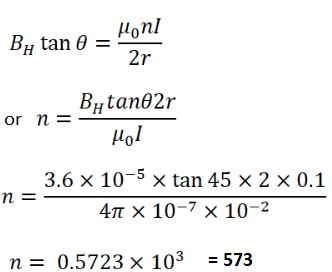Question 17: A moving-coil galvanometer has a 50-turn coil of size 2 cm × 2 cm. It is suspended between the magnetic poles producing a magnetic field of 0.5 T. Find the torque on the coil due to the magnetic field when a current of 20 mA passes through it.

Solution:

Area of the cross section of the coil = A = 4 cm2

Magnetic field strength due to the presence of the pole = B = 0.5 T

n = number of turns = 50

B = 0.5 T and I = 20mA = 20 X 10-3

Let τ = torque experienced by coil placed in an external magnetic field:

τ = ni (A x B) [Here A and B are vectors]

τ = ni AB sin90o

τ = 50 x 20 x 10-3 x 4 x 10-4 x 0.5 = 2×10-4 N-m

Question 18: A short magnet produces a deflection of 37° in a deflection magnetometer in Tan-A position when placed at a separation of 10 cm from the needle. Find the ratio of the magnetic moment of the magnet to the earth’s horizontal magnetic field.

Solution:

Let M = magnetic moment of the magnet and BH = earth’s horizontal magnetic field.

By magnetometer theory, we have

for short magnet,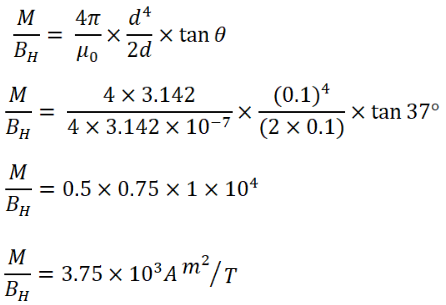Question 19: The magnetometer of the previous problem is used with the same magnet in Tan-B position. Where should the magnet be placed to produces a 37° deflection of the needle?

Solution:

Here, d = 10 cm = 0.1 m

For short magnet, θ = 37°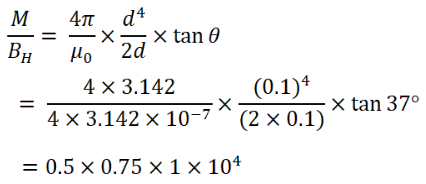From the magnetometer theory in Tan-B position:

M/BH = 3.75 x 103 Am2/T …(1)

When l is very very small than d,Question 20: A deflection magnetometer is placed with its arms in north-south direction. How and where should a short magnet having M/BH = 40 A m2T–1 be placed so that the needle can stay in any position?

Solution:

Given: M/BH = 40 A m2T–1

We know,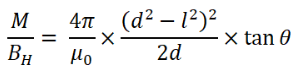For d >>>>>>>> l

M/BH = 4π /μ0 x d3/2

40 = [4×3.142]/[ 4×3.142×10-7] x d3/2

Or d = 0.02 m = 2 cm

Question 21: A bar magnet takes π/10 second to complete one oscillation in an oscillation magnetometer. The moment of inertia of the magnet about the axis of rotation is 1.2 × 10–4 kg m2 and the earth’s horizontal magnetic field is 30 μT. Find the magnetic moment of the magnet.

Solution:

Given: T = π/10 second

BH = 30 μT = 30 x 10-6 T

Moment of inertia = I = 1.2 × 10–4 kg m2

Time period of a magnetometer: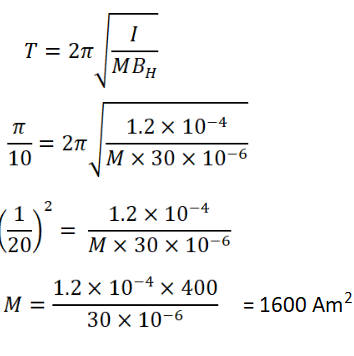Question 22: The combination of two bar magnets makes 10 oscillations per second in an oscillation magnetometer when like poles are tied together and 2 oscillations per second when unlike poles are tied together. Find the ratio of the magnetic moments of the magnets. Neglect any induced magnetism.

Solution:

Let f1 and f2 be the number of oscillations per second made by the combination of bar magnets with unlike poles and like poles,

f1 = 10 s-1 and f2 = 2 s-1

The frequency of oscillations in the magnetometer,

f = 1/2π x √[MBH/i]

When unlike poles are tied together, the effective magnetic moment = M= M1 + M2

When like poles are tied together, the effective magnetic moment = M= M1 – M2

Now,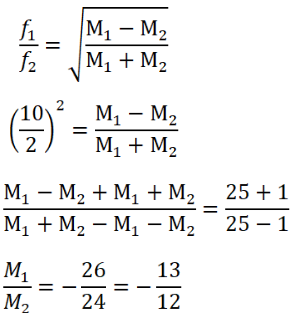Question 23: A short magnet oscillates in an oscillation magnetometer with a time period of 0.10 s where the earth’s horizontal magnetic field is 24 μT. A downward current of 18 A is established in a vertical wire placed 20 cm east of the magnet. Find the new time period.

Solution:

Time period of oscillation = T1 = 0.10 sec

and BH = 24μT = 24 x 10-6 T

Distance of wire from the magnet = d = 20cm = 0.2m

Downward current in the vertical wire = I = 18A

When a wire (carrying current) is placed near the magnet, the effective magnetic field changes.

Now, the net magnetic field can be obtained by subtracting the magnetic field from the earth’s magnetic field due to the wire.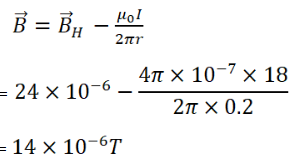Let T be the time period of the coil. As “T” is inversely proportional to magnetic field:

T1/T2 = √[B/BH]

where, T1 and T2 be the time periods of the coil in the absence of the wire and in the presence the wire respectively.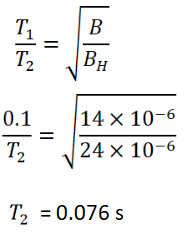Question 24: A bar magnet makes 40 oscillations per minute in an oscillation magnetometer. An identical magnet is demagnetized completely and is placed over the magnet in the magnetometer. Find the time taken for 40 oscillations by this combination. Neglect any induced magnetism.

Solution:

Let T1 = 1/40 min

Find T2

Given: I’ = 2I

We know, T1/T2 = √[I/I’]

=> 1/40T2 = √(1/2)

T2 = 1/800

or T2 = 0.03536 min

Now,

Time taken for 1 oscillation = 0.03536 min

Time taken for 40 oscillation = 40×0.03536 = 1.414 = 2min

Question 25: A short magnet makes 40 oscillations per minute when used in an oscillation magnetometer at a place where the earth’s horizontal magnetic field is 25 μT. Another short magnet of magnetic moment 1.6 A m2 is placed 20 cm east of the oscillating magnet. Find the new frequency of oscillation if the magnet has its north pole

(a) towards north and

(b) towards south.

Solution:

Let v1 = 40 oscillation/min

BH = 25 μT

(a) towards north

Let v2 be the new velocity.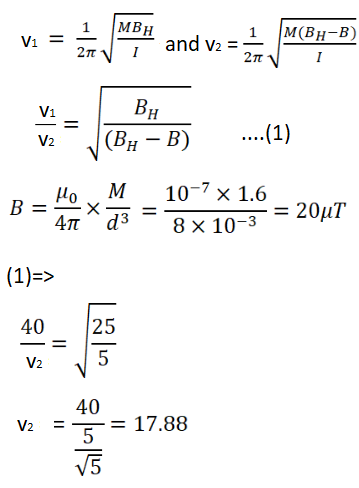(b) towards south: